# Kauffman polynomial

An invariant of oriented links (cf. also Knot theory).

It is a Laurent polynomial of two variables associated to ambient isotopy classes of links in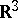(or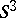), constructed by L. Kauffman in 1985 and denoted by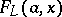(cf. also Isotopy).

The construction starts from the invariant of non-oriented link diagrams (cf. also Knot and link diagrams),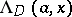. For a diagram of a trivial link ofcomponents,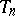, put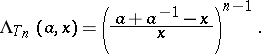The Kauffman skein quadruple satisfies a skein relation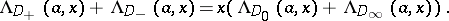Furthermore, the second and the third Reidemeister moves (cf. also Knot and link diagrams) preserve the invariant, while the first Reidemeister move is changing it byor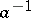(depending on whether the move is positive or negative). To define the Kauffman polynomial from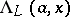one considers an oriented link diagram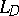, represented by, and puts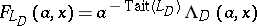, where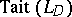is the Tait (or writhe) number of an oriented link diagram(cf. also Writhing number).

The Jones polynomial and its-cable version are special cases of the Kauffman polynomial.

There are several examples of different links with the same Kauffman polynomial. In particular, the knot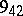and its mirror image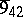are different but have the same Kauffman polynomial. Some other examples deal with mutant and their generalizations:-rotor constructions,-cables of mutants and any satellites of connected sums (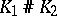and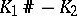). The following two questions are open (1998) and of great interest:

1) Is there a non-trivial knot with Kauffman polynomial equal to?

2) Is there infinite number of different knots with the same Kauffman polynomial?

The number of Fox-colourings can be computed from the Kauffman polynomial (at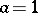,). Kauffman constructed his polynomial building on his interpretation of the Jones–Conway (HOMFLYpt), the Brandt–Lickorish–Millett and the Ho polynomials.

The important feature of the Kauffman polynomial is its computational complexity (with respect to the number of crossings of the diagram; cf. also Complexity theory). It is conjectured to be exponential and it is proven to be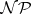-hard (cf. also); so, up to the conjecture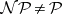, the Kauffman polynomial cannot be computed in polynomial time. The complexity of computing the Alexander polynomial is, in contrast, polynomial. The Kauffman polynomial is independent from the Alexander polynomial, it often distinguishes a knot from its mirror image but, for example, it does not distinguish the knots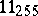and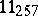(in Perko's notation), but the Alexander polynomial does distinguish these knots. The Kauffman polynomials are stratified by the Vassiliev invariants, which have polynomial-time computational complexity.

If one considers the skein relation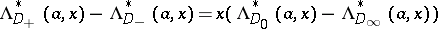, one gets the Dubrovnik polynomial, which is a variant of the Kauffman polynomial.

The Kauffman polynomial leads to the Kauffman skein module of-manifolds.